## 3D Transformations in Computer Graphics-

We have discussed-

• Transformation is a process of modifying and re-positioning the existing graphics.
• 3D Transformations take place in a three dimensional plane.

In computer graphics, various transformation techniques are-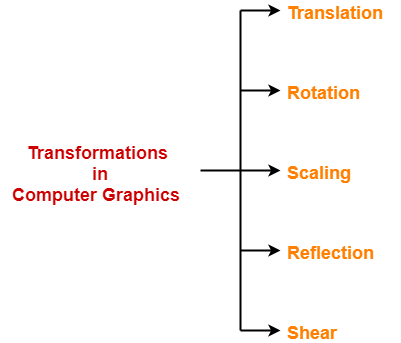## 3D Reflection in Computer Graphics-

• Reflection is a kind of rotation where the angle of rotation is 180 degree.
• The reflected object is always formed on the other side of mirror.
• The size of reflected object is same as the size of original object.

Consider a point object O has to be reflected in a 3D plane.

Let-

• Initial coordinates of the object O = (Xold, Yold, Zold)
• New coordinates of the reflected object O after reflection = (Xnew, Ynew,Znew)

In 3 dimensions, there are 3 possible types of reflection-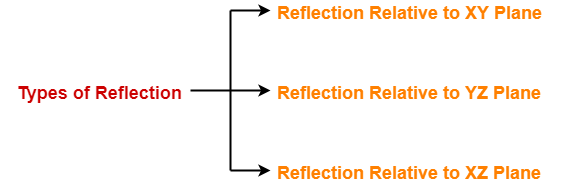• Reflection relative to XY plane
• Reflection relative to YZ plane
• Reflection relative to XZ plane

### Reflection Relative to XY Plane:

This reflection is achieved by using the following reflection equations-

• Xnew = Xold
• Ynew = Yold
• Znew = -Zold

In Matrix form, the above reflection equations may be represented as-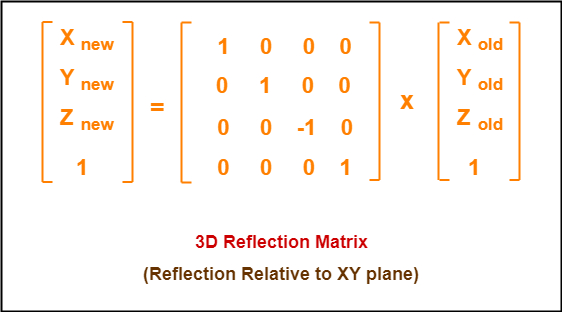### Reflection Relative to YZ Plane:

This reflection is achieved by using the following reflection equations-

• Xnew = -Xold
• Ynew = Yold
• Znew = Zold

In Matrix form, the above reflection equations may be represented as-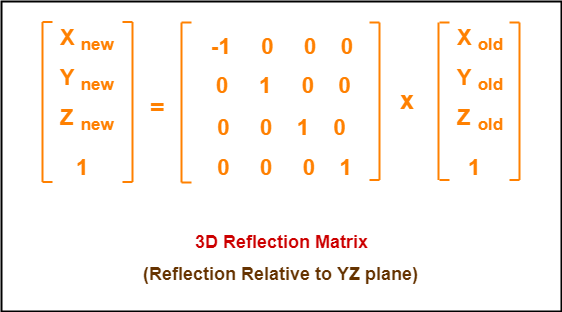### Reflection Relative to XZ Plane:

This reflection is achieved by using the following reflection equations-

• Xnew = Xold
• Ynew = -Yold
• Znew = Zold

In Matrix form, the above reflection equations may be represented as-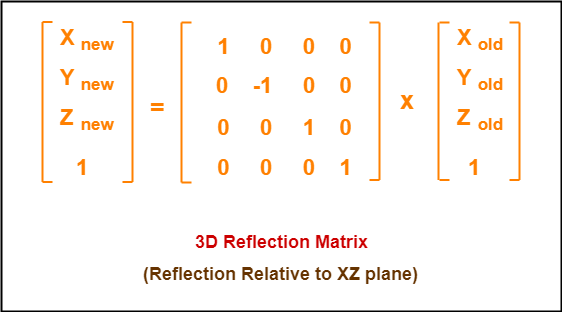## Problem-01:

Given a 3D triangle with coordinate points A(3, 4, 1), B(6, 4, 2), C(5, 6, 3). Apply the reflection on the XY plane and find out the new coordinates of the object.

## Solution-

Given-

• Old corner coordinates of the triangle = A (3, 4, 1), B(6, 4, 2), C(5, 6, 3)
• Reflection has to be taken on the XY plane

### For Coordinates A(3, 4, 1)

Let the new coordinates of corner A after reflection = (Xnew, Ynew, Znew).

Applying the reflection equations, we have-

• Xnew = Xold = 3
• Ynew = Yold = 4
• Znew = -Zold = -1

Thus, New coordinates of corner A after reflection = (3, 4, -1).

### For Coordinates B(6, 4, 2)

Let the new coordinates of corner B after reflection = (Xnew, Ynew, Znew).

Applying the reflection equations, we have-

• Xnew = Xold = 6
• Ynew = Yold = 4
• Znew = -Zold = -2

Thus, New coordinates of corner B after reflection = (6, 4, -2).

### For Coordinates C(5, 6, 3)

Let the new coordinates of corner C after reflection = (Xnew, Ynew, Znew).

Applying the reflection equations, we have-

• Xnew = Xold = 5
• Ynew = Yold = 6
• Znew = -Zold = -3

Thus, New coordinates of corner C after reflection = (5, 6, -3).

Thus, New coordinates of the triangle after reflection = A (3, 4, -1), B(6, 4, -2), C(5, 6, -3).

## Problem-02:

Given a 3D triangle with coordinate points A(3, 4, 1), B(6, 4, 2), C(5, 6, 3). Apply the reflection on the XZ plane and find out the new coordinates of the object.

## Solution-

Given-

• Old corner coordinates of the triangle = A (3, 4, 1), B(6, 4, 2), C(5, 6, 3)
• Reflection has to be taken on the XZ plane

### For Coordinates A(3, 4, 1)

Let the new coordinates of corner A after reflection = (Xnew, Ynew, Znew).

Applying the reflection equations, we have-

• Xnew = Xold = 3
• Ynew = -Yold = -4
• Znew = Zold = 1

Thus, New coordinates of corner A after reflection = (3, -4, 1).

### For Coordinates B(6, 4, 2)

Let the new coordinates of corner B after reflection = (Xnew, Ynew, Znew).

Applying the reflection equations, we have-

• Xnew = Xold = 6
• Ynew = -Yold = -4
• Znew = Zold = 2

Thus, New coordinates of corner B after reflection = (6, -4, 2).

### For Coordinates C(5, 6, 3)

Let the new coordinates of corner C after reflection = (Xnew, Ynew, Znew).

Applying the reflection equations, we have-

• Xnew = Xold = 5
• Ynew = -Yold = -6
• Znew = Zold = 3

Thus, New coordinates of corner C after reflection = (5, -6, 3).

Thus, New coordinates of the triangle after reflection = A (3, -4, 1), B(6, -4, 2), C(5, -6, 3).

To gain better understanding about 3D Reflection in Computer Graphics,

Watch this Video Lecture

Next Article- 3D Shearing in Computer Graphics

Get more notes and other study material of Computer Graphics.

Watch video lectures by visiting our YouTube channel LearnVidFun.

## 2D Transformations in Computer Graphics-

We have discussed-

• Transformation is a process of modifying and re-positioning the existing graphics.
• 2D Transformations take place in a two dimensional plane.

In computer graphics, various transformation techniques are-## 2D Reflection in Computer Graphics-

• Reflection is a kind of rotation where the angle of rotation is 180 degree.
• The reflected object is always formed on the other side of mirror.
• The size of reflected object is same as the size of original object.

Consider a point object O has to be reflected in a 2D plane.

Let-

• Initial coordinates of the object O = (Xold, Yold)
• New coordinates of the reflected object O after reflection = (Xnew, Ynew)

### Reflection On X-Axis:

This reflection is achieved by using the following reflection equations-

• Xnew = Xold
• Ynew = -Yold

In Matrix form, the above reflection equations may be represented as-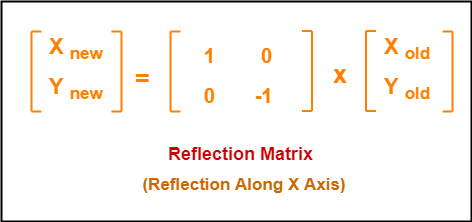For homogeneous coordinates, the above reflection matrix may be represented as a 3 x 3 matrix as-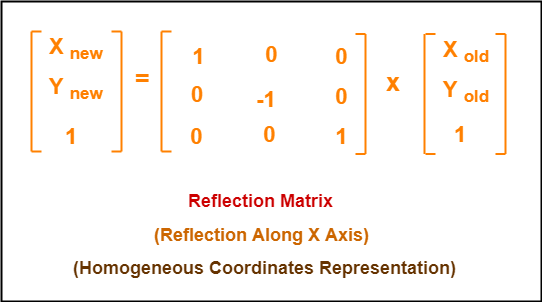### Reflection On Y-Axis:

This reflection is achieved by using the following reflection equations-

• Xnew = -Xold
• Ynew = Yold

In Matrix form, the above reflection equations may be represented as-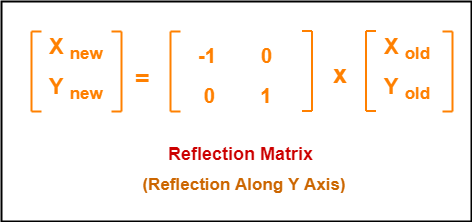For homogeneous coordinates, the above reflection matrix may be represented as a 3 x 3 matrix as-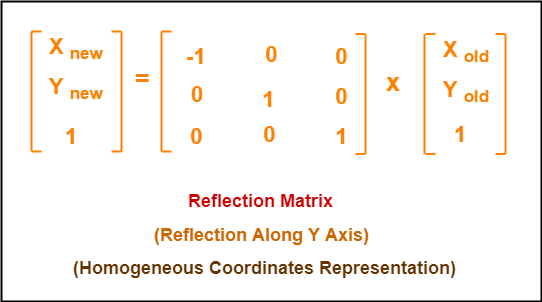## Problem-01:

Given a triangle with coordinate points A(3, 4), B(6, 4), C(5, 6). Apply the reflection on the X axis and obtain the new coordinates of the object.

## Solution-

Given-

• Old corner coordinates of the triangle = A (3, 4), B(6, 4), C(5, 6)
• Reflection has to be taken on the X axis

### For Coordinates A(3, 4)

Let the new coordinates of corner A after reflection = (Xnew, Ynew).

Applying the reflection equations, we have-

• Xnew = Xold = 3
• Ynew = -Yold = -4

Thus, New coordinates of corner A after reflection = (3, -4).

### For Coordinates B(6, 4)

Let the new coordinates of corner B after reflection = (Xnew, Ynew).

Applying the reflection equations, we have-

• Xnew = Xold = 6
• Ynew = -Yold = -4

Thus, New coordinates of corner B after reflection = (6, -4).

### For Coordinates C(5, 6)

Let the new coordinates of corner C after reflection = (Xnew, Ynew).

Applying the reflection equations, we have-

• Xnew = Xold = 5
• Ynew = -Yold = -6

Thus, New coordinates of corner C after reflection = (5, -6).

Thus, New coordinates of the triangle after reflection = A (3, -4), B(6, -4), C(5, -6).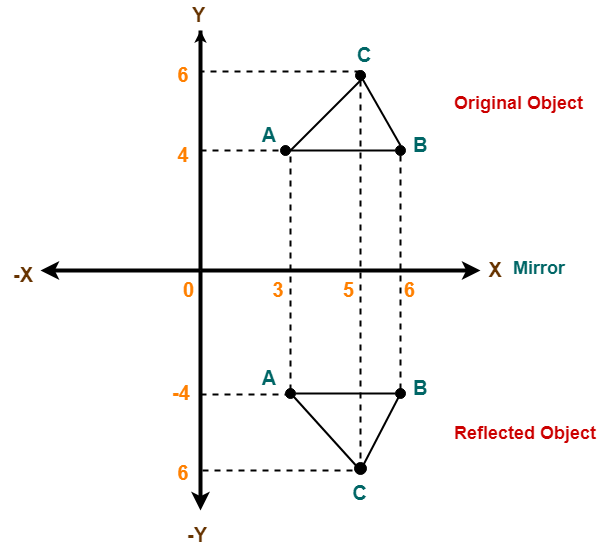## Problem-02:

Given a triangle with coordinate points A(3, 4), B(6, 4), C(5, 6). Apply the reflection on the Y axis and obtain the new coordinates of the object.

## Solution-

Given-

• Old corner coordinates of the triangle = A (3, 4), B(6, 4), C(5, 6)
• Reflection has to be taken on the Y axis

### For Coordinates A(3, 4)

Let the new coordinates of corner A after reflection = (Xnew, Ynew).

Applying the reflection equations, we have-

• Xnew = -Xold = -3
• Ynew = Yold = 4

Thus, New coordinates of corner A after reflection = (-3, 4).

### For Coordinates B(6, 4)

Let the new coordinates of corner B after reflection = (Xnew, Ynew).

Applying the reflection equations, we have-

• Xnew = -Xold = -6
• Ynew = Yold = 4

Thus, New coordinates of corner B after reflection = (-6, 4).

### For Coordinates C(5, 6)

Let the new coordinates of corner C after reflection = (Xnew, Ynew).

Applying the reflection equations, we have-

• Xnew = -Xold = -5
• Ynew = Yold = 6

Thus, New coordinates of corner C after reflection = (-5, 6).

Thus, New coordinates of the triangle after reflection = A (-3, 4), B(-6, 4), C(-5, 6).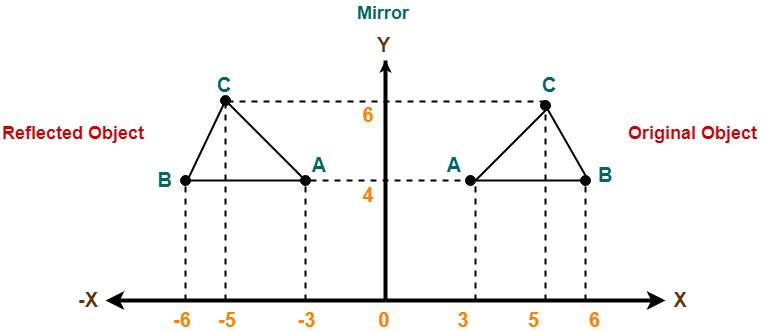To gain better understanding about 2D Reflection in Computer Graphics,

Watch this Video Lecture

Next Article- 2D Shearing in Computer Graphics

Get more notes and other study material of Computer Graphics.

Watch video lectures by visiting our YouTube channel LearnVidFun.